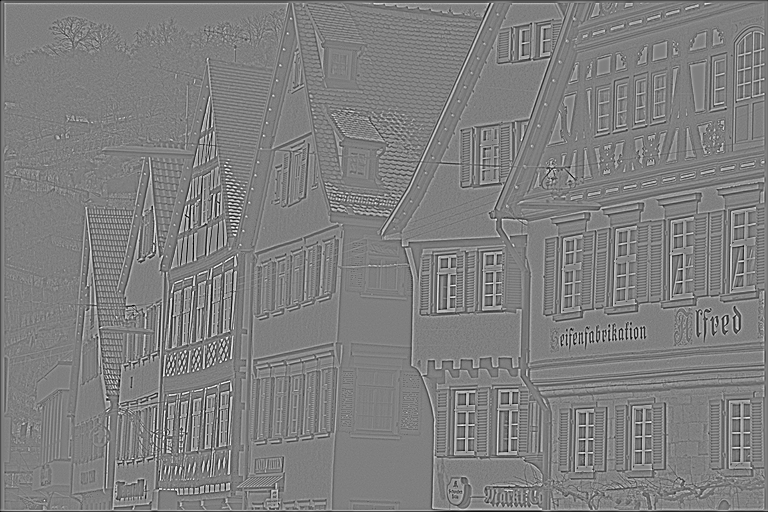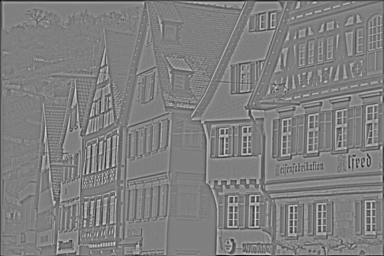VPI - Vision Programming Interface

1.1 Release

Laplacian Pyramid Generator

Overview

A Laplacian pyramid is an image representation consisting of a set of band-pass images and a low-frequency residual.

Applications range from image compression to detail manipulation, where the input image is decomposed into frequency bands represented as a Laplacian pyramid. Each band can be manipulated independently. The final image can be reconstructed by summing up all bands and the low-frequency residual.

Input Gaussian Pyramid Output (optional) Laplacian Pyramid OutputImplementation

VPI implements an approximated Laplacian pyramid as a difference of Gaussian pyramids, as shown below:

Laplacian Pyramid algorithm high-level implementation

The kth level of Laplacian pyramid can be obtained by the following formula:

$L_k(I) = G_k(I) - u(G_{k+1}(I))$

Where:

• $$I$$ is the input image.
• $$L_k(I)$$ is the kth level of Laplacian pyramid.
• $$G_k(I)$$ is the kth level of Gaussian pyramid.
• $$u(\bullet)$$ is a 2x scale-up operation.

The algorithm repeats until all levels are generated.

The VPI implementation optionally returns the intermediate Gaussian pyramid used in computation, in case this representation is also needed. There is no performance penalty in doing so.

Usage

Language:
1. Import VPI module
import vpi
2. (optional) Create a 4-level VPI pyramid that will store the gaussian pyramid by-product. It uses the VPI image input to get its dimensions and format.
gaussian = vpi.Pyramid(input.size, input.format, 4)
3. Returns the 4-level Laplacian pyramid created from the input image using the CUDA backend. It also returns the corresponding gaussian pyramid. Input is a VPI image, and outputs are VPI pyramids.
with vpi.Backend.CUDA:
output = input.laplacian_pyramid(4, out_gaussian=gaussian)
1. Initialization phase:
1. Include the header that defines the Laplacian pyramid generator function:
Declares functions that handle Laplacian pyramids.
2. Define the input image object:
VPIImage input = /*...*/;
struct VPIImageImpl * VPIImage
A handle to an image.
Definition: Types.h:215
3. Create the output pyramid with the desired number of levels (in this example 4) and scale factor (fixed at 0.5):
int32_t w, h;
vpiImageGetSize(input, &w, &h);
VPIPyramid output;
vpiPyramidCreate(w, h, VPI_IMAGE_FORMAT_F32, 4, 0.5, 0, &output);
@ VPI_IMAGE_FORMAT_F32
Single plane with one 32-bit floating point channel.
Definition: ImageFormat.h:122
VPIStatus vpiImageGetSize(VPIImage img, int32_t *width, int32_t *height)
Get the image size in pixels.
struct VPIPyramidImpl * VPIPyramid
A handle to an image pyramid.
Definition: Types.h:221
VPIStatus vpiPyramidCreate(int32_t width, int32_t height, VPIImageFormat fmt, int32_t numLevels, float scale, uint32_t flags, VPIPyramid *pyr)
Create an empty image pyramid instance with the specified flags.
4. Optionally, create the Gaussian pyramid with the same dimensions as the Laplacian pyramid output. Its image format must be the same of the input image:
vpiImageGetFormat(input, &type);
VPIPyramid gaussianPyr;
vpiPyramidCreate(w, h, type, 4, 0.5, 0, &gaussianPyr);
VPIImageFormat
Pre-defined image formats.
Definition: ImageFormat.h:99
VPIStatus vpiImageGetFormat(VPIImage img, VPIImageFormat *format)
Get the image format.
5. Create the stream to which the algorithm is to be submitted for execution:
VPIStream stream;
vpiStreamCreate(0, &stream);
struct VPIStreamImpl * VPIStream
A handle to a stream.
Definition: Types.h:209
VPIStatus vpiStreamCreate(uint32_t flags, VPIStream *stream)
Create a stream instance.
2. Processing phase:
1. Submit the algorithm to the stream, along with the input image, output pyramid, and optional Gaussian pyramid. The algorithm is executed by the CPU backend:
VPIStatus vpiSubmitLaplacianPyramidGenerator(VPIStream stream, uint32_t backend, VPIImage input, VPIPyramid output, VPIPyramid gaussianPyr, VPIBorderExtension border)
Computes the Laplacian pyramid from the input image.
@ VPI_BACKEND_CPU
CPU backend.
Definition: Types.h:92
@ VPI_BORDER_CLAMP
Border pixels are repeated indefinitely.
Definition: Types.h:238
2. Optionally, wait until the processing is done:
vpiStreamSync(stream);
VPIStatus vpiStreamSync(VPIStream stream)
Blocks the calling thread until all submitted commands in this stream queue are done (queue is empty)...
3. Cleanup phase:
1. Free resources held by the stream, the input image, the output pyramid, and the optional Gaussian pyramid:
vpiPyramidDestroy(gaussianPyr);
void vpiImageDestroy(VPIImage img)
Destroy an image instance.
void vpiPyramidDestroy(VPIPyramid pyr)
Destroy an image pyramid instance as well as all resources it owns.
void vpiStreamDestroy(VPIStream stream)
Destroy a stream instance and deallocate all HW resources.

For more information, see Laplacian Pyramid Generator in the "API Reference" section of VPI - Vision Programming Interface.

Limitations and Constraints

CPU and CUDA backends

• The input image and pyramid's base level must have the same dimensions.
• Only scale=0.5 is supported (i.e. only dyadic pyramids can be generated).
• If a Gaussian pyramid is used, its image must have the same format as the input image.
• The following image formats are accepted for input image:
• The following image formats are accepted for an output Laplacian pyramid:
• The coarsest level of the Laplacian pyramid is equivalent in concept to that of the Gaussian pyramid. However, in cases where the Laplacian pyramid output format has less positive dynamic range than the input format, i.e. the input format is VPI_IMAGE_FORMAT_U8 and the output format is VPI_IMAGE_FORMAT_S8 or U16 and S16, the pixel values of the output in the coarsest level are divided by 2 to avoid overflow.

Other backends

• Not supported.

Performance

For information on how to use the performance table below, see Algorithm Performance Tables.
Before comparing measurements, consult Comparing Algorithm Elapsed Times.
For further information on how performance was benchmarked, see Performance Benchmark.

-